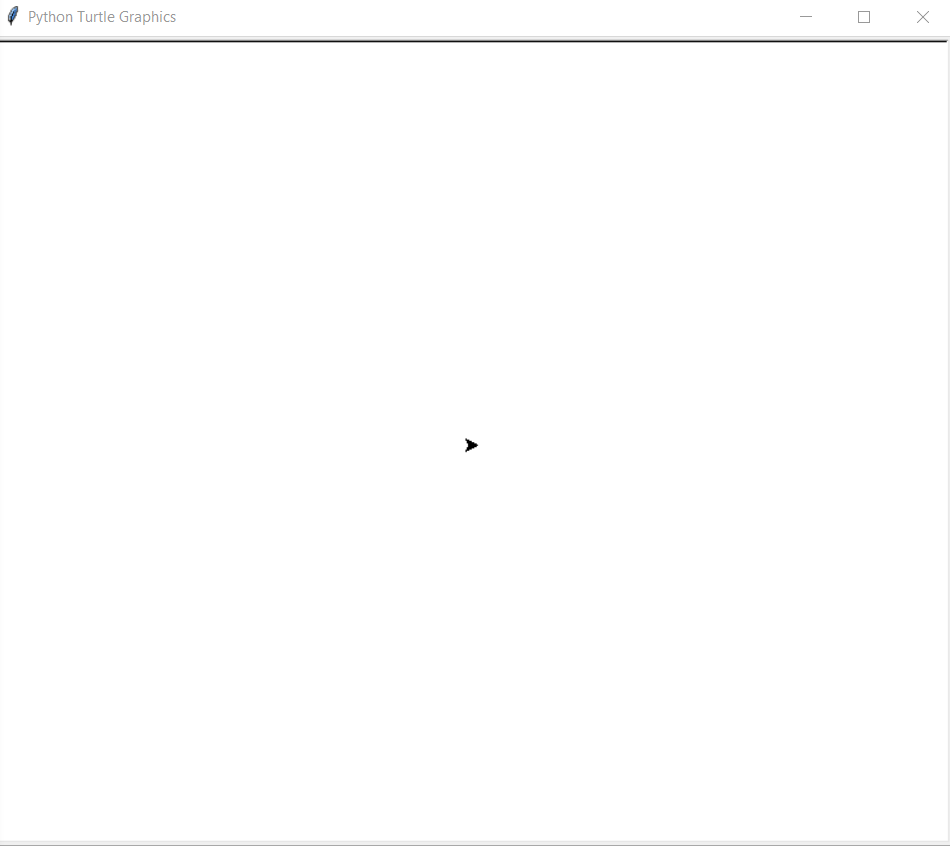# Python – Draw Octagonal shape Using Turtle Graphics

In this article, we will learn how to Make an Octagon using Turtle Graphics in Python. For that lets first know what is Turtle Graphics.

### Turtle graphics

• backward(length): moves the pen in the backward direction by x unit.
• right(angle): rotate the pen in the clockwise direction by an angle x.
• left(angle): rotate the pen in the anticlockwise direction by an angle x.
• penup(): stop drawing of the turtle pen.
• pendown(): start drawing of the turtle pen.

## Approach

• Import the turtle modules.
• Get a screen to draw on
• Define an instance for the turtle.
• For a drawing, an Octagon executes a loop 8 times.
• In every iteration move the turtle 100 units forward and move it left 45 degrees( corresponding to 135 degrees between two sides, so 180-135=45 degrees).
• This will make up an angle of 135 degrees between 2 sides.
• 8 iterations will make up an Octagon perfectly.
Below is the Python implementation of the above approach:

## Python3

 `# import for turtle module` `import` `turtle`   `# making a workScreen` `ws ``=` `turtle.Screen()`   `# defining a turtle instance` `geekyTurtle ``=` `turtle.Turtle()`   `# iterating the loop 8 times` `for` `i ``in` `range``(``8``):` `  `  `    ``# moving turtle 100 units forward` `    ``geekyTurtle.forward(``100``)` `    `  `    ``# turning turtle 45 degrees so ` `    ``# as to make perfect angle for an octagon` `    ``geekyTurtle.left(``45``)`

Output:Octagon

Whether you're preparing for your first job interview or aiming to upskill in this ever-evolving tech landscape, GeeksforGeeks Courses are your key to success. We provide top-quality content at affordable prices, all geared towards accelerating your growth in a time-bound manner. Join the millions we've already empowered, and we're here to do the same for you. Don't miss out - check it out now!